## Solutions of Reflection of Light Lakhmir Singh Manjit Kaur VSAQ, SAQ, MCQ, HOTS, and LAQ Pg No. 189 Class 10 Physics1. For what position of an object, a concave mirror forms a real image equal in size to the object?

→ At the centre of curvature, concave mirror forms a real image equal in size to the object.

2. Where should an object be placed in front of the concave mirror so as to obtain its virtual, erect and magnified image?

→ Object should be placed between pole and focus in front of the concave mirror so as to obtain its virtual, erect and magnified image.

3. For which positions of the object does a concave mirror produce an inverted, magnified and real image?

→ Object should be placed between focus and center of curvature to produce an inverted, magnified and real image.

4. If an object is placed at the focus of a concave mirror, where is the image formed?

→ If an object is placed at the focus of a concave mirror, the image is formed at infinity.

5. If an object is at infinity (very large distance) in front of a concave mirror, where is the image formed?

→ The image is formed at focus, if an object is at infinity (very large distance) in front of a concave mirror.

6. For what position of an object, a real and diminished image is formed by a concave mirror?

→ A real and diminished image is formed by a concave mirror when object is placed beyond center of curvature.

7. Copy this figure in your answer book and show the direction of the light ray after reflection:Direction of light ray after reflection is like this.

11. Which kind of mirror is used in the headlights of a car? Why is it used for this purpose?

→ Concave mirror is used in the headlights of a car.

It is used in headlights as when bulb is placed at the focus of a concave mirror , then diverging light ray is reflected to produce a strong, parallel side beam of light.

12. Explain why, a ray of light passing through the centre of curvature of a concave mirror gets reflected back along the same path.

→ When a ray of light passes through the centre of curvature of a concave mirror gets reflected back along the same path as it falls normally (perpendicularly) on the mirror surface; angle of reflection is 0° and the angle of incidence is also 0°.

13. What is the minimum number of rays required for locating the image formed by a concave mirror for an object? Draw a ray diagram to show the formation of a virtual image by a concave mirror.

→ Minimum two rays are required.
Ray diagram for the formation of a virtual image by a concave mirror:14. With the help of a ray diagram, determine the position, nature and size of the image formed of an object placed at the centre of curvature of a concave mirror.→ When an object is placed at the centre of curvature of a concave mirror, the image formed is at the centre of curvature, is real and inverted and is of same size as the object.

15. Describe with the help of a diagram, the nature, size and position of the image formed when an object is placed beyond the centre of curvature of a concave mirror.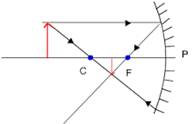→ When an object is placed beyond the centre of curvature (C) of a concave mirror, the image is between the focus and centre of curvature, is real and inverted and is smaller than the object (or diminished).

16. If an object is placed at a distance of 8 cm from a concave mirror of focal length 10 cm, discuss the nature of the image formed by drawing the ray diagram.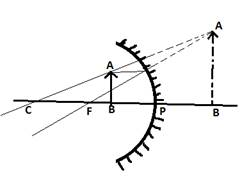The focal length of the mirror is PF = 10 cm.
The object is placed at B such that
Object distance, PB = 8 cm.
This means that the object lies between the pole and focus of the concave mirror.
The image formed is virtual, erect and magnified behind the mirror.

17. Draw a ray diagram showing how a concave mirror can be used to produce a real, inverted and diminished image of an object.18. Which mirror is used as a torch reflector ? Draw a labelled diagram to show how a torch reflector can be used to produce a parallel beam of light. Where is the bulb placed in relation to the torch reflector?

→ For a torch reflector concave mirror is used.Bulb is placed at the focus of the torch reflector.

19. State where an object must be placed so that the image formed by a concave mirror is:
(a) erect and virtual.
(b) at infinity.
(c) the same size as the object.

→ (a) an object must be placed Between pole and focus of the mirror so that the image formed by a concave mirror is erect and virtual.
(b) an object must be placed At the focus of the mirror so that the image formed by a concave mirror is at infinity.
(c) an object must be placed At the centre of curvature of the mirror so that the image formed by a concave mirror is the same size as the object.

20. With the help of a labelled ray diagram, describe how a converging mirror can be used to give an enlarged upright image of an object.Converging mirror can be used to give an enlarged upright image of an object by placing the object between focus (F) and pole (P) of the concave mirror.

21. Make labeled ray diagrams to illustrate the formation of :
(a) a real image by a converging mirror.
(b) a virtual image by a converging mirror.
Mark clearly the pole, focus, centre of curvature and position of object in each case.

→ (a) A real image by a converging mirror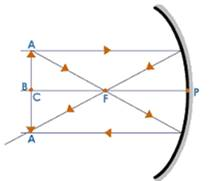(b) A virtual image by a converging mirror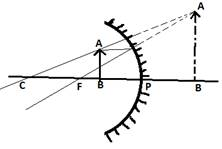22. Briefly describe how you would find the focal length of a concave mirror quickly but approximately.

→ To find the focal length of a concave mirror quickly but approximately we can apply few practical skills
1) When image is formed at focus that means object is at considerable distance.
2) Sharp image is formed when object is at focus such that focal length is equal to object distance.

23. Which type of mirror is used in a solar furnace? Support your answer with reason.

→ Concave mirror is used in a solar furnace.24. Name the type of mirror used by dentists. How does it help ?

→ Concave mirror is used by dentists.
Since the tooth looks much bigger through concave mirror hence it becomes easy to examine the defect in the tooth.

25. Explain why, concave mirrors are used as shaving mirrors.

→ Concave mirrors are used as shaving mirrors as when the face is placed close to a concave mirror the concave mirror produces a magnified and erect image of the face so that it becomes easier to make a smooth shave.

26. Give two uses of concave mirrors. Explain why you would choose concave mirrors for these uses.

→ Uses of concave mirror
(i) Concave mirror are used by dentists. Since the tooth looks much bigger through concave mirror hence it becomes easy to examine the defect in the tooth.
(ii) Concave mirrors are used as shaving mirrors as when the face is placed close to a concave mirror the concave mirror produces a magnified and erect image of the face so that it becomes easier to make a smooth shave.

27A. Draw ray-diagrams to show the formation of images when the object is placed in front of a concave mirror (converging mirror):
(i) between its pole and focus
(ii) between its centre of curvature and focus
Describe the nature, size and position of the image formed in each case.

→ (i) Nature: Virtual, erect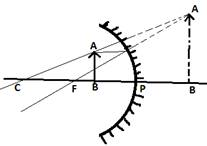Size: magnified
Position: Behind the mirror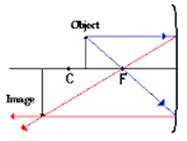(ii) Nature: real, inverted
Size: magnified

Position: on the same side as object.

27B. State one use of concave mirror based on the formation of image as in case (i) above.

→ Concave mirror are used by dentists. Since the tooth looks much bigger through concave mirror hence it becomes easy to examine the defect in the tooth.

28A. Give two circumstances in which a concave mirror can form a magnified image of an object placed in front of it. Illustrate your answer by drawing labelled ray diagrams for both.

→ (i) When the object is placed between the pole and focus of a concave mirror, a magnified image is formed.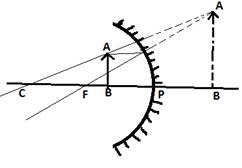(ii) When the object is placed between the focus and the centre of curvature of a concave mirror, a magnified image is formed.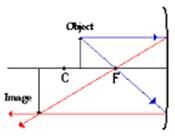28B. Which one of these circumstances enables a concave mirror to be used as a shaving mirror?

→ A concave mirror can be used as a shaving mirror when the object is placed between the pole and focus of a concave mirror.

Multiple Choice Questions (MCQs)-Pg-190

29. The real image formed by a concave mirror is larger than the object when the object is :
A. between focus and centre of curvature.
B. distance less than the focal length
C. curvature at a distance equal to radius of curvature
D. at a distance greater than radius of curvature30. The real image formed by a concave mirror is smaller than the object if the object is :
A. between centre of curvature and focus
B. at a distance equal to focal length
C. at a distance equal to radius of curvature
D. at a distance greater than radius of curvature31. The image formed by a concave mirror is virtual, erect and magnified. The position of object is :
A. between pole and focus
B. between focus and centre of curvature
C. at pole
D. at focus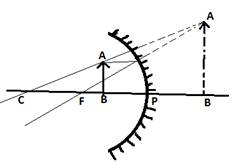32. The image formed by a concave mirror is real, inverted and of the same size as the object. The position of the object must then be:
A. at the focus
B. between the centre of curvature and focus
C. beyond the centre of curvature
D. at the centre of curvature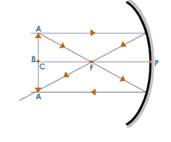33. The image formed by a concave mirror is real, inverted and highly diminished (much smaller than the object). The object must be:
A. between pole and focus
B. at infinity
C. at the centre of curvature
D. at focus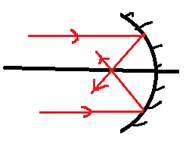34. The angle of incidence for a ray of light passing through the centre of curvature of a concave mirror is:
A. 45°
B. 0°
C. 90°
D. 180°35. In the concave reflector of a torch, the bulb is placed:
A. at the focus of reflector
B. between the pole and focus of reflector
C. between focus and centre of curvature of reflector
D. at the centre of curvature of reflector36. The focal length of a small concave mirror is 2.5 cm. In order to use this concave mirror as a dentist's mirror, the distance of tooth from the mirror should be :
A. 1.5 cm
B. 2.5 cm
C. 4.5 cm
D. 3.5 cm

→ Focal length = 2.5 cm
To use as dentist mirror the image should be bigger and thus more than f.

Questions Based on High Order Thinking Skills (HOTS)-Pg-191

37. An object is 200 mm in front of a concave mirror which produces an upright image (erect image). The radius of curvature of the mirror is:
A) less than 200 mm
B) between 200 mm and 400 mm
C) exactly 400 mm
D) more than 400 mm

→ (D) Since the image formed is upright, the object lies within the focus of the concave mirror.
So, f > 200 mm
We know R = 2f
So, R > 400 mm
i.e. Radius of curvature is more than 400 mm.

38. A virtual, erect and magnified image of an object is to be produced with a concave mirror of focal length 14 Cm. Which of the following object distance should be chosen for this purpose ?
(i) 10 Cm (ii) 15 Cm (iii) 20 Cm

→ 10 cm, as image formed is virtual, erect and magnified if the object is placed between the pole and focus of the concave mirror only.

39. A concave mirror has a focal length of 22 Cm. At which of the following distance should a person hold his face from this concave mirror so that it may act as a shaving mirror ?
(a) 45 cm
(b) 20 cm
(c) 25 cm
(d) 30 cm

→ 20 cm, the image formed is erect and magnified only if the object is placed between the pole and focus of the concave mirror.

40. An object is placed at the following distances from a concave mirror of focal length 16 cm, turn by turn:
(a) 36 cm
(b) 32 cm
(c) 21 cm
(d) 9 cm
Which position of the object will produce:
(i) a magnified real image?
(ii) a magnified virtual image?
(iii) a diminished real image?
(iv) an image of same size as the object?

→ (i) A magnified real image - 21 cm - When the object is placed between focus and centre of curvature.
(ii) A magnified virtual image - 9 cm -When the object is placed within its focus.
(iii) A diminished real image - 36 cm -When the object is placed beyond the centre of curvature.
(iv) An image of same size as the object - 32 cm -When the object is at the centre of curvature.

1. According to the "New Cartesian Sign Convention" for mirrors, what sign has been given to the focal length of:
(i) a concave mirror?
(ii) a convex mirror ?

→ (i) According to the "New Cartesian Sign Convention" for mirrors, Negative sign has been given to the focal length of a concave mirror.
(ii) According to the "New Cartesian Sign Convention" for mirrors, Positive sign has been given to the focal length of a convex mirror.

2. Which type of mirror has :
(a) positive focal length?
(b) negative focal length?

→ (a) Convex mirror has positive focal length.
(b) Concave mirror has negative focal length.

3. What is the nature of a mirror having a focal length of, +10 cm ?

→ The nature of a mirror having a focal length of, +10 cm is Convex mirror.

4. What kind of mirror can have a focal length of, - 20 cm ?

→ Concave mirror has a focal length of - 20 cm.

5. Complete the following sentence:
All the ______ are measured from the Pole of a spherical mirror.

→ Distances

6. What sign (+ve or -ve) has been given to the following on the basis of Cartesian Sign Convention?
(a) Height of a real image.
(b) Height of a virtual image.

→ (a) sign (+ve or -ve) that has been given to the Height of a real image. on the basis of Cartesian Sign Convention is Negative.
(b) sign (+ve or -ve) that has been given to the Height of a virtual image on the basis of Cartesian Sign Convention is Positive.

7. Describe the New Cartesian Sign Convention used in optics. Draw a labelled diagram to illustrate this is convention.

→ According to the New Cartesian Sign Convention:
(i) All the distances are measured from pole of the mirror.
(ii) Distances measured in the same direction as that of incident light are taken as positive.
(iii) Distances measured against the direction of incident light are taken as negative.
(iv) Distances measured upward and perpendicular to the principal axis are taken as positive.
(v) Distance measured downward and perpendicular to the principal axis are taken as negative.

8. Giving reasons, state the 'signs' (positive or negative) which can be given to the following:
(a) object distance (u) for a concave mirror or convex mirror
(b) image distances (v) for a concave mirror
(c) image distances (v) for a convex mirror

→ (a) According to sign convention, distances measured against the direction of incident light are taken as negative such that Object distance (u) for a concave mirror or convex mirror is always negative.
(b) According to sign convention, distances measured in the same direction as that of incident light are taken as positive and distances measured against the direction of incident light are taken as negative such that if the image is formed on the left side of the mirror, then the image distance (v) will be negative and if the image is formed on the right side of the mirror, then the image distance (v) will be positive.
(c) According to sign convention, distances measured in the same direction as that of incident light are taken as positive such that Image distances (v) for a convex mirror is always positive.

Multiple Choice Questions (MCQs)-Pg-193

9. According to New Cartesian Sign Convention:
A. focal length of concave mirror is positive and that of convex mirror is negative.
B. focal length of concave mirror is negative and that of convex mirror is positive.
C. focal length of both concave and convex mirrors is negative.
D. focal length of both concave and convex mirrors is positive.10. One of the following does not apply to a concave mirror. This is:
A. focal length is positive
B. image distance can be positive or negative
C. image distance is always negative
D. height of image can be positive or negative1. If the magnification of a body of size 1 m is 4, what is the size of the image ?2. What is the position of the image when an object is placed at a distance of 20 cm from a concave mirror of focal length 30 cm ?

→ Object distance, u = -20 cm
Focal length, f = -30 cm (concave mirror)
Image distance, v =?
We know that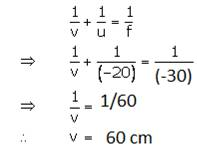3. What is the nature of image formed by a concave mirror if the magnification produced by the mirror is
a) + 4, and (b) - 2 ?

→ (a) the nature of image formed by a concave mirror is Virtual and erect.
(b) the nature of image formed by a concave mirror is Real and inverted.

4. State the relation between object distance, image distance and focal length of a spherical mirror (concave mirror or convex mirror).5. Write the mirror formula. Give the meaning of each symbol which occurs in it.

→ Mirror formula is given below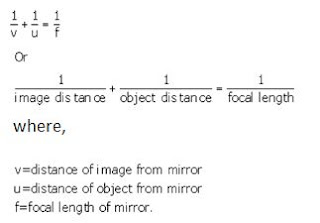6. What is the ratio of the height of an image to the height of an object known as ?

→ Magnification.

7. Define linear magnification produced by a mirror.

→ The ratio of the height of image to the height of object is known as linear magnification.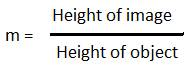8. Write down a formula for the magnification produced by a concave mirror.
(a) in terms of height of object and height of image
(b) in terms of object distance and image distance

→ (a) Magnification produced by a concave mirror in terms of height of object and height of image is(b) Magnification produced by a concave mirror in terms of height of object and height of image iswhere, h2= height of image
h1= height of object
v= image distance
u= object distance

9. Describe the nature of image formed when the object is placed at a distance of 20 cm from a concave mirror of focal length 15 cm.

→ Here, u = - 20 cm; f = -15 cm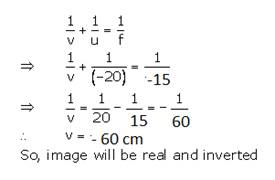10. Fill in the following blanks with suitable words :
(a) If the magnification has a ______ sign, then image is virtual and erect
(b) If the magnification has a ______ sign, then the image is real and inverted.

→ (a) plus
(b) minus

11. An object is placed at a distance of 10 cm from a concave mirror of focal length 15cm.
(a) Draw a ray diagram for the formation of image.
(b) Calculate the image distance.
(c) State two characteristics of the image formed.(b) f=-15cm, u=-10cm, v=?
We know that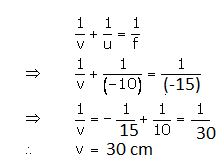(c) Characteristics of image formed is virtual and erect.

12. If an object of 10 cm height is placed at a distance of 24 cm from a concave mirror of focal length 12 cm, find the position, nature and height of the image.

→ h1 = 10cm, u = -24cm, f = -12cm
We know that
1/v +1/u = 1/f ⇒ 1/v + 1/(-24)= 1/(-12)⇒ 1/v =(-1/24)⇒ v = -24 cm
m  =  h2 / h1= - v / u = -(-24)/(-24)= -1
⇒ h2/h1 = -1
⇒ h2 = -1×  h1
⇒ h2= - 10 cm
the image formed will be of same height at a distance of 24 cm from the mirror and would be inverted in nature.

13. At what distance from a concave mirror of focal length 10 cm should an object 2 cm long be placed in order to get an erect image 6 cm tall ?

→ f = (-10cm)
h= 2cm
h= 6cm (erect image)
u = ?
We know that: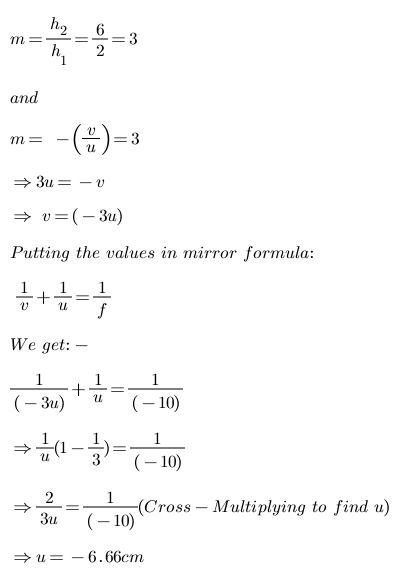14. When an object is placed at a distance of 15 cm from a concave mirror, its image is formed at 10 cm in front of the mirror. Calculate the radius of curvature of the mirror.

→ u= -15cm, v= -10cm
f=?
We know thatRadius of curvature = 2f = 12 cm.

15. An object 3 cm high is placed at a distance of 8 cm from a concave mirror which produces a virtual image 4.5 cm high:
(i) What is the focal length of the mirror?
(ii) What is the position of image?
(iii) Draw a ray-diagram to show the formation of image.

→ given
Height of the object h1=3 cm,
distance between object and mirror  u=-8 cm, Height of the image h2=6 cm (virtual image)

Formula used:where,M is the magnification of the image H2 is the height of the image
H1 is the height of the object
v is the distance between image and mirror u is the distance between object and mirrorF is the focal length of the mirror v is the distance between the image and the mirror u is the distance between the object and mirror putting the value in equation(1)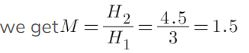This means that the image will form at a distance of  12 cm behind the mirror i.e. virtual image is formed Putting the value in equation(2), we getf= -24 cm the focal length of the convex mirror used is 24 cm the Ray diagram is16. A converging mirror forms a real image of height 4 cm of an object of height 2 cm placed 20 cm away from the mirror:
(i) Calculate the image distance.
(ii) What is the focal length of the mirror?